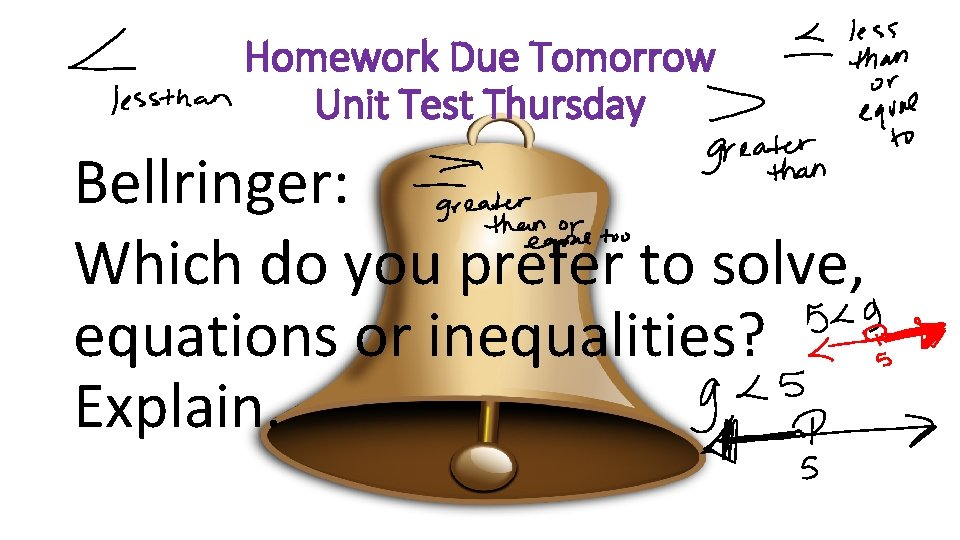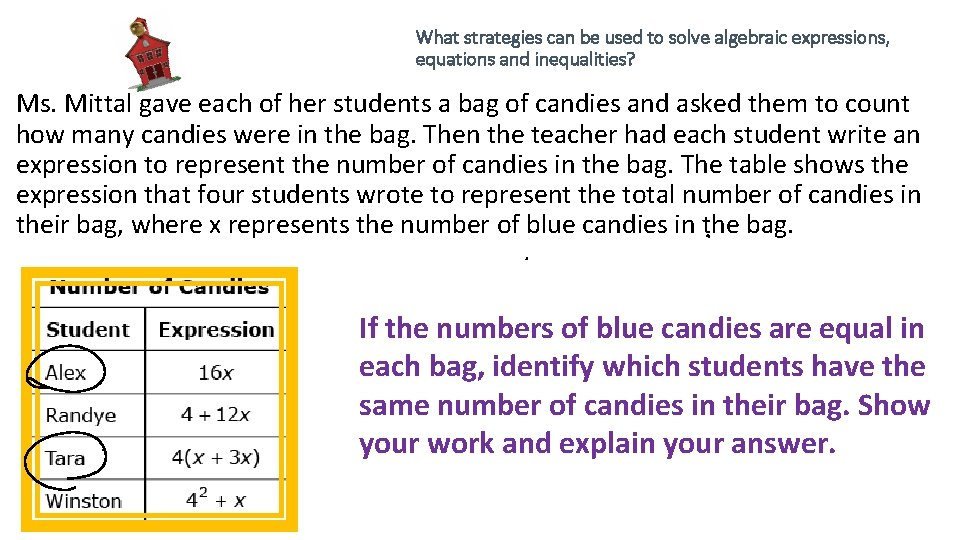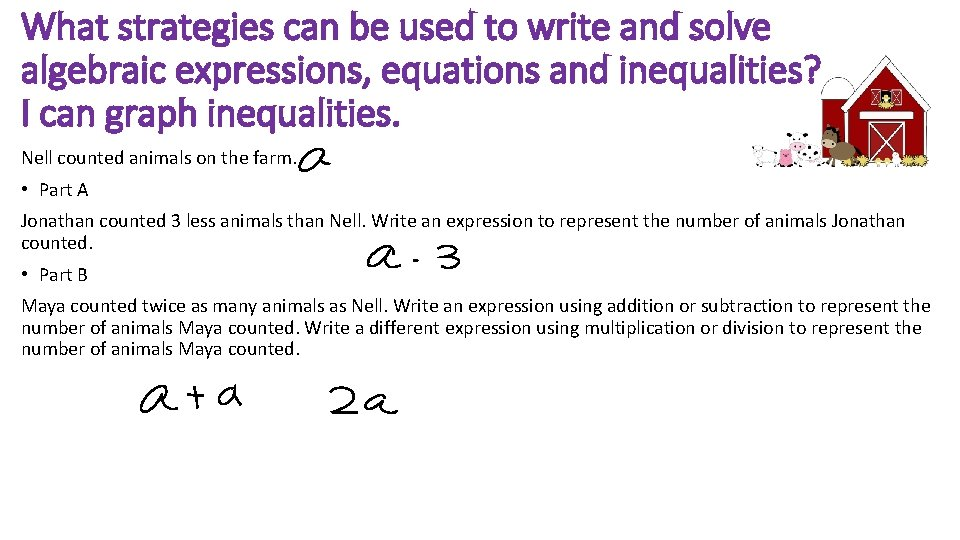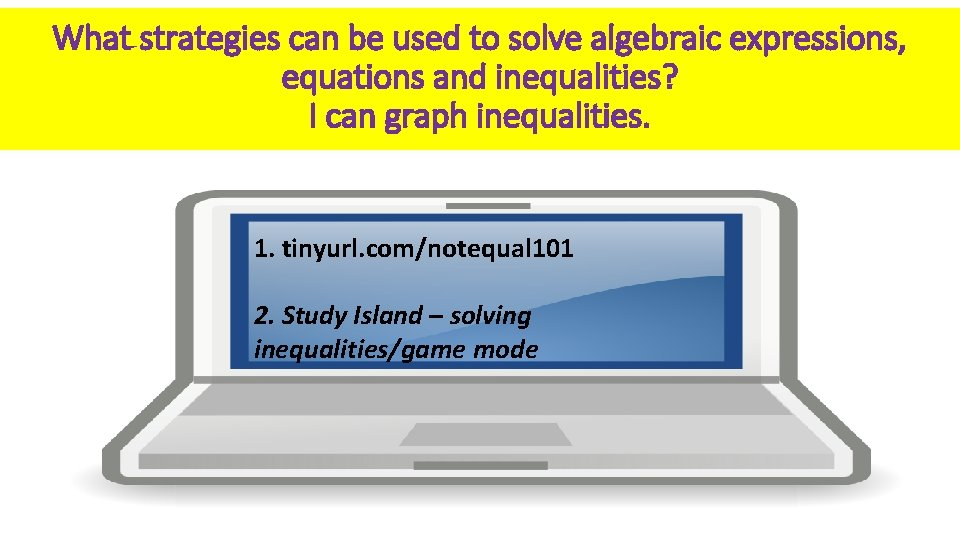# Homework Due Tomorrow Unit Test Thursday Bellringer Which

• Slides: 5Homework Due Tomorrow Unit Test Thursday Bellringer: Which do you prefer to solve, equations or inequalities? Explain.What strategies can be used to solve algebraic expressions, equations and inequalities? Ms. Mittal gave each of her students a bag of candies and asked them to count how many candies were in the bag. Then the teacher had each student write an expression to represent the number of candies in the bag. The table shows the expression that four students wrote to represent the total number of candies in their bag, where x represents the number of blue candies in the bag. If the numbers of blue candies are equal in each bag, identify which students have the same number of candies in their bag. Show your work and explain your answer.What strategies can be used to solve algebraic expressions, equations and inequalities? Ms. Mittal gave each of her students a bag of candies and asked them to count how many candies were in the bag. Then the teacher had each student write an expression to represent the number of candies in the bag. The table shows the expression that four students wrote to represent the total number of candies in their bag, where x represents the number of blue candies in the bag. Alex has 48 candies in her bag. Using the table, write an equation that shows how to use x to find the number of blue candies in Alex's bag. Solve for x. Show your workWhat strategies can be used to write and solve algebraic expressions, equations and inequalities? I can graph inequalities. Nell counted animals on the farm. • Part A Jonathan counted 3 less animals than Nell. Write an expression to represent the number of animals Jonathan counted. • Part B Maya counted twice as many animals as Nell. Write an expression using addition or subtraction to represent the number of animals Maya counted. Write a different expression using multiplication or division to represent the number of animals Maya counted.What strategies can be used to solve algebraic expressions, equations and inequalities? I can graph inequalities. 1. tinyurl. com/notequal 101 2. Study Island – solving inequalities/game mode